Worked example of calculating F-statistics from genotypic data:
 Genotype AA Aa aa Subpopulation 1 125 250 125 Subpopulation 2 50 30 20 Subpopulation 3 100 500 400

N (number of individuals genotyped. The sum of each of the rows in the table above):

Population 1:   500
Population 2:   100
Population 3:   1,000
Remember that the number of alleles is TWICE the number of genotypes.

Step 1.  Calculate the gene (allele) frequencies:

Each homozygote will have two alleles, each heterozygote will have one allele.  Note that the denominator will be twice Ni (twice as many alleles as individuals).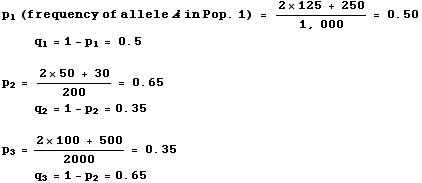Eqns FST.1

Step 2. Calculate the expected genotypic counts under Hardy-Weinberg Equilibrium, and then calculate the excess or deficiency of homozygotes in each subpopulation.

Pop. 1        Expected AA = 500*0.52                    = 125     (= observed)

Expected Aa =  500*2*0.5*0.5           = 250     (= observed)

Expected aa =  500*0.52                      = 125     (= observed)

Pop. 2        Expected AA = 100*0.652                   = 42.25  (observed has excess of 7.75)

Expected Aa =  100*2*0.65*0.35        =  45.5   (observed has deficit of 15.5)

Expected aa =  100*0.352                      = 12.25  (observed has excess of 7.75)

Note that sum of two types of homozygote excess = amount of heterozygote deficiency.
These quantities have to balance (it's a mathematical necessity, given that p + q =1.

Pop. 3        Expected AA = 1,000*0.352                   = 122.5  (observed has deficiency of 22.5)

Expected Aa =  1,000*2*0.65*0.35        =  455   (observed has excess of 45)

Expected aa =  1,000*0.352                      = 422.5  (observed has deficiency of 22.5)

Summary of homozygote deficiency or excess relative to HWE:

Pop. 1.  Observed = Expected: perfect fit
Pop. 2.  Excess of 15.5 homozygotes: some inbreeding
Pop. 3.  Deficiency of 45 homozygotes: outbred or experiencing a Wahlund effect (isolate breaking).

Step 3. Calculate the local observed heterozygosities of each subpopulation (we will call them Hobs s, where the s subscript refers to the sth of n populations -- 3 in this example).  Here we count genotypes:

Hobs 1 = 250 / 500                = 0.5

Hobs 2 = 30 / 100                               = 0.3

Hobs 3 = 500 / 1000                                          = 0.5

Step 4.  Calculate the local expected heterozygosity, or gene diversity, of each subpopulation (modified version of Eqn 35.1):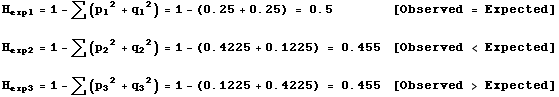Eqns FST.2

(With two alleles it would actually be easier to use 2pq than to use the summation format of Eqn 33.1)

Notation: Note that I am using p1 and q1 here (where the subscripts refer to subpopulations 1 through 3).  We would need to use multiple subscripts if we were using the notation of Eqn 35.1 where the alleles are pi  (and the i refer to alleles 1 to k).  Indeed, with real multi-locus multipopulation data, we would have a triple summation and three subscripts;  one for alleles (i =1 to k), one for the loci ( l =1 to m), and one for subpopulations (s = 1 to n).
Step 5. Calculate the local inbreeding coefficient of each subpopulation (same as Eqn 35.4, except that we are subscripting for the subpopulations):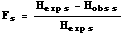where s (s = 1 to 3) refers to the subpopulation                Eqn FST.3
F1 = (0.5 — 0.5) / 0.5             = 0

F2 = (0.455 — 0.3) / 0.455     = 0.341
[positive F means fewer heterozygotes than expected indicates inbreeding]

F3 = (0.455 — 0.5) / 0.455     = -0.099
[negative F means more heterozygotes than expected means excess outbreeding]

Step 6. Calculate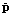(p-bar, the frequency of allele A) over the total population.
[Note that if we had more alleles we could put this and Step 7 all together as a single "global gene frequencies" step, or have one for each allele frequency].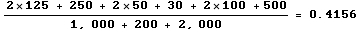{genotype splitting method}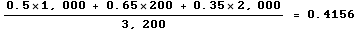{using Eqn FST.1 values for ps}

Note that we weight by population size

Step 7. Calculate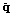(q-bar, the frequency of allele a) over the total population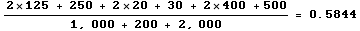Check: p-bar + q-bar =  0.4156 + 0.5844 = 1.0 (as required by Eqn 31.1).

The check doesn't guarantee that our result is correct, but if they don't sum to one, we know we miscalculated.

Step 8.   Calculate the global heterozygosity indices (over Individuals, Subpopulations and Total population)

Note that the first two calculations employ a weighted average of the values in the whole set of subpopulations.

HI based on observed heterozygosities in individuals in subpopulations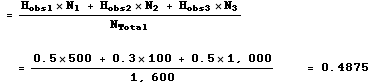Eqn FST.4

HS based on expected heterozygosities in subpopulations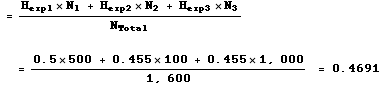Eqn FST.5

HT based on expected heterozygosities for overall total population (using global allele frequencies and a modified form of Eqn 35.1):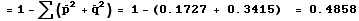Eqn FST.6

or we could calculate it as      2*p-bar *q-bar   = 2 * 0.4156 * 0.5844  = 0.4858

Step 9.  CALCULATE THE GLOBAL  F-STATISTICS:
Compare and contrast the global FISbelow with the "local inbreeding coefficient" Fs of Step 5.
Here we are using a weighted average of the individual heterozygosities over all the subpopulations.
Both FIS and  Fs are, however, based on the observed heterozygosities,
whereas FST and FIT are based on expected heterozygosities.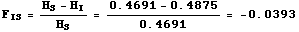Eqn FST.7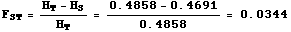Eqn FST.8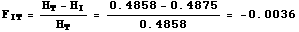Eqn FST.9

Notation note: the subscripts I, S, and T are not summation subscripts.  They simply indicate the level of our analysis.  Likewise, the s on Fs in Step 5 or on the ps in Step 1 (the s was implicit there) just tell us what we are referring to.  In contrast, the subscripts for Eqn 35.1 and 35.2 are used in summations and change as we work through the pieces of the calculation.

Step 10.  Finally, draw some conclusions about the genetic structure of the population and its subpopulations.
1)  One of the possible HWE conclusions we could make:
Pop. 1 is consistent with HWE (results of Step 2)
2)  Two of the possible "local inbreeding" conclusions we could make from Step 5:
Pop. 2 is inbred (results of Step 5), and
Pop. 3 may have disassortative mating or be experiencing a Wahlund effect (more heterozygotes than expected).
3)  Conclusion concerning overall degree of genetic differentiation (FST)
Subdivision of populations, possibly due to genetic drift,
accounts for approx. 3.4% of the total genetic variation
(result of Eqn FST.8 FST calculation in Step 9),
4)  No excess or deficiency of heterozygotes over the total population (FIT  is nearly zero).